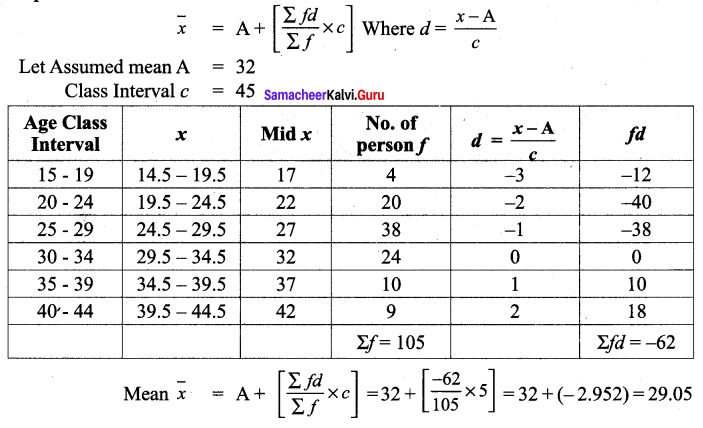# Samacheer Kalvi 9th Maths Solutions Chapter 8 Statistics Ex 8.1

## Tamilnadu Samacheer Kalvi 9th Maths Chapter 8 Solutions Statistics Ex 8.1

Question 1.
In a week, temperature of a certain place is measured during winter are as follows 26°C, 24°C, 28°C, 31°C, 30°C, 26°C, 24°C. Find the mean temperature of the week.
Solution: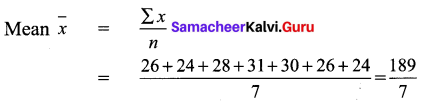Mean temperature of the week = 27°CQuestion 2.
The mean weight of 4 members of a family is 60kg. Three of them have the weight 56kg, 68kg and 72kg respectively. Find the weight of the fourth member.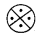Solution: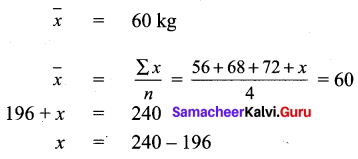∴ The weight of the fourth member = 44 kg

Question 3.
In a class test in mathematics, 10 students scored 75 marks, 12 students scored 60 marks, 8 students scored 40 marks and 3 students scored 30 marks. Find the mean of their score.
Solution:
Total number of students = 10 + 12 + 8 + 3 = 33
Total score of 33 students = 10 × 75 + 12 × 60 + 8 × 40 + 3 × 30
= 750 + 720 + 320 + 90 = 1880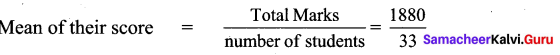= 56.96 or 57 approximately

Question 4.
In a research laboratory scientists treated 6 mice with lung cancer using natural medicine. Ten days later, they measured the volume of the tumor in each mouse and given the results in the table.Find the mean.
Solution: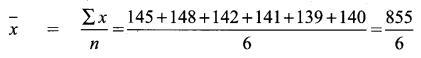x = 142.5 mm3

Question 5.
If the mean of the following data is 20.2, then find the value of pSolution: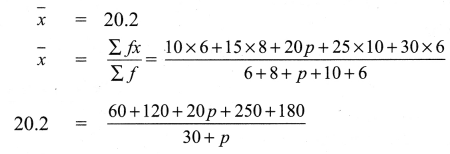(30 +p) 20.2 = 610+20p
606 +20.2p = 610+20p
20.2 p – 20p = 610 – 606 = 4
0.2 = 4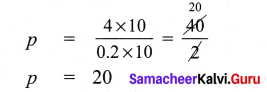Question 6.
In the class, weight of students is measured for the class records. Calculate mean weight of the class students using Direct method.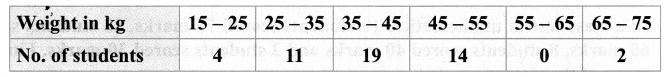Solution: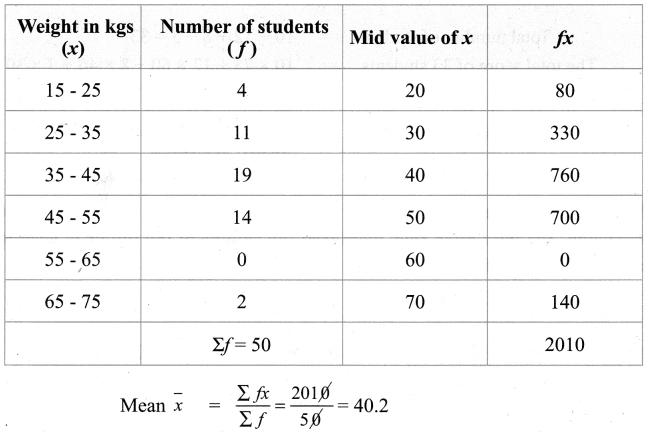Question 7.
Calculate the mean of the following distribution using Assumed Mean Method: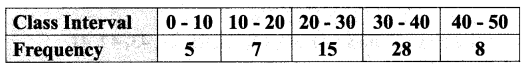Solution:
Let Assumed mean be 25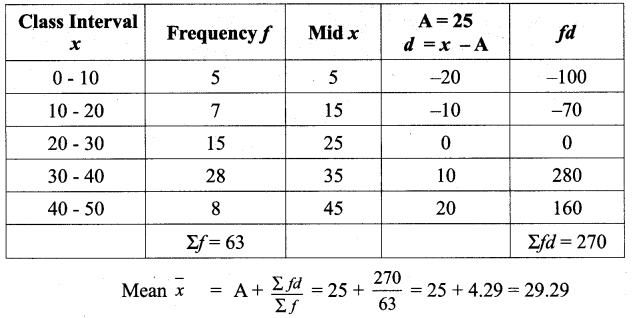Question 8.
Find the Arithmetic Mean of the following data using Step Deviation Method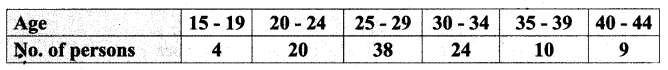Solution:
Standard Deviation Method